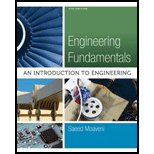# For the cooling of steel plates discussed in Section 18.4 (Figure 18.17) using linear interpolation, estimate the temperature of the plate at time equal to 1 hr, from the temperature data at 0.8 hr and 1.2 hour. Compare the estimated temperature value to the actual value of 308° C. What is the percentage of error?### Engineering Fundamentals: An Intro...

5th Edition
Saeed Moaveni
Publisher: Cengage Learning
ISBN: 9781305084766

#### Solutions

Chapter
Section### Engineering Fundamentals: An Intro...

5th Edition
Saeed Moaveni
Publisher: Cengage Learning
ISBN: 9781305084766
Chapter 18, Problem 32P
Textbook Problem
126 views

## For the cooling of steel plates discussed in Section 18.4 (Figure 18.17) using linear interpolation, estimate the temperature of the plate at time equal to 1 hr, from the temperature data at 0.8 hr and 1.2 hour. Compare the estimated temperature value to the actual value of 308° C. What is the percentage of error?

To determine

Estimate the temperature of plate at 1hr and the percentage of error.

### Explanation of Solution

Given data:

Refer to Figure 18.17 in section 18.4 in textbook,

The value of temperature at 0.8hr is 379°C.

The actual value of temperature at 1hr is 308°C.

The value of temperature at 1.2hr is 252°C.

Formula used:

Formula to calculate the error is,

%error=estimated valueactual valueactual value×100% . (1)

Calculation:

Refer to Figure 18.17 in textbook, the graph shows the relationship between temperature and time.

To find the value of temperature at 1hr use the neighboring values of temperature at 0.8hr and 1.2hr.

Therefore, to find the temperature at 1hr the Figure 18.17 is shown as Figure 1 as follows:

From the Figure 1, draw the two similar triangles ACE and BCD to find the altitude using a linear interpolation manner as shown Figure 2.

Using similar angle theorem,

BCAC=BDAE (2)

Refer to Figure 2,

The values for BC,AC,BDandAE are calculated as follows,

BC=1.2hr1hr=0.2hr

AC=1.2hr0.8hr=0.4hr

AE=379°C252°C=127°C

BD=T1hr252°C

Substitute 0.2hr for BC, 0.4hr for AC, 127°C for AE, and T1hr252°C for BD in equation (2),

0

### Still sussing out bartleby?

Check out a sample textbook solution.

See a sample solution

#### The Solution to Your Study Problems

Bartleby provides explanations to thousands of textbook problems written by our experts, many with advanced degrees!

Get Started

Find more solutions based on key concepts
What tool is used to true and dress an aluminum oxide wheel?

Precision Machining Technology (MindTap Course List)

What is a table, and what role does it play in the relational model?

Database Systems: Design, Implementation, & Management

Identify and briefly describe five common fact-finding methods.

Systems Analysis and Design (Shelly Cashman Series) (MindTap Course List)

The force system shown can be replaced with a single, equivalent couple CR. Determine CR.

International Edition---engineering Mechanics: Statics, 4th Edition

What advantages does SAS have over SCSI? (380)

Enhanced Discovering Computers 2017 (Shelly Cashman Series) (MindTap Course List)

Voltage can be described as ____________ .

Welding: Principles and Applications (MindTap Course List)

If your motherboard supports ECC DDR3 memory, can you substitute non-ECC DDR3 memory?

A+ Guide to Hardware (Standalone Book) (MindTap Course List)